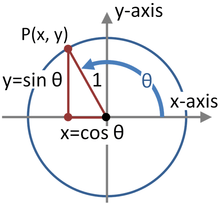# Identity (mathematics)

Not to be confused with Identity element or Identity function.Visual proof of the Pythagorean identity. For any angle θ, The point (cos(θ),sin(θ)) lies on the unit circle, which satisfies the equation x2+y2=1. Thus, cos2(θ)+sin2(θ)=1.

In mathematics an identity is an equality relation A = B, such that A and B contain some variables and A and B produce the same value as each other regardless of what values (usually numbers) are substituted for the variables. In other words, A = B is an identity if A and B define the same functions. This means that an identity is an equality between functions that are differently defined. For example, (a + b)2  =  a2 + 2ab + b2 and cos2(x) + sin2(x) = 1 are identities. Identities are sometimes indicated by the triple bar symbol instead of =, the equals sign.

## Common identities

### Trigonometric identities

Geometrically, these are identities involving certain functions of one or more angles. They are distinct from triangle identities, which are identities involving both angles and side lengths of a triangle. Only the former are covered in this article.

These identities are useful whenever expressions involving trigonometric functions need to be simplified. An important application is the integration of non-trigonometric functions: a common technique involves first using the substitution rule with a trigonometric function, and then simplifying the resulting integral with a trigonometric identity.

One example iswhich is true for all complex values of(since the complex numbersare the domain of sin and cos), as opposed towhich is true only for some values of, not all. For example, the latter equation is true whenfalse when.

### Exponential identities

Main article: Exponentiation

The following identities hold for all integer exponents, provided that the base is non-zero:Exponentiation is not commutative. This contrasts with addition and multiplication, which are. For example, 2 + 3 = 3 + 2 = 5 and 2 · 3 = 3 · 2 = 6, but 23 = 8, whereas 32 = 9.

Exponentiation is not associative either. Addition and multiplication are. For example, (2 + 3) + 4 = 2 + (3 + 4) = 9 and (2 · 3) · 4 = 2 · (3 · 4) = 24, but 23 to the 4 is 84 or 4,096, whereas 2 to the 34 is 281 or 2,417,851,639,229,258,349,412,352. Without parentheses to modify the order of calculation, by convention the order is top-down, not bottom-up:### Logarithmic identities

Several important formulas, sometimes called logarithmic identities or log laws, relate logarithms to one another.

#### Product, quotient, power and root

The logarithm of a product is the sum of the logarithms of the numbers being multiplied; the logarithm of the ratio of two numbers is the difference of the logarithms. The logarithm of the p-th power of a number is p times the logarithm of the number itself; the logarithm of a p-th root is the logarithm of the number divided by p. The following table lists these identities with examples. Each of the identities can be derived after substitution of the logarithm definitions x = blogb(x), and/or y = blogb(y), in the left hand sides.

Formula Example
productquotientpowerroot#### Change of base

The logarithm logb(x) can be computed from the logarithms of x and b with respect to an arbitrary base k using the following formula:Typical scientific calculators calculate the logarithms to bases 10 and e. Logarithms with respect to any base b can be determined using either of these two logarithms by the previous formula:Given a number x and its logarithm logb(x) to an unknown base b, the base is given by:### Hyperbolic function identities

Main article: Hyperbolic function

The hyperbolic functions satisfy many identities, all of them similar in form to the trigonometric identities. In fact, Osborn's rule states that one can convert any trigonometric identity into a hyperbolic identity by expanding it completely in terms of integral powers of sines and cosines, changing sine to sinh and cosine to cosh, and switching the sign of every term which contains a product of 2, 6, 10, 14, ... sinhs.

The Gudermannian function gives a direct relationship between the circular functions and the hyperbolic ones that does not involve complex numbers.